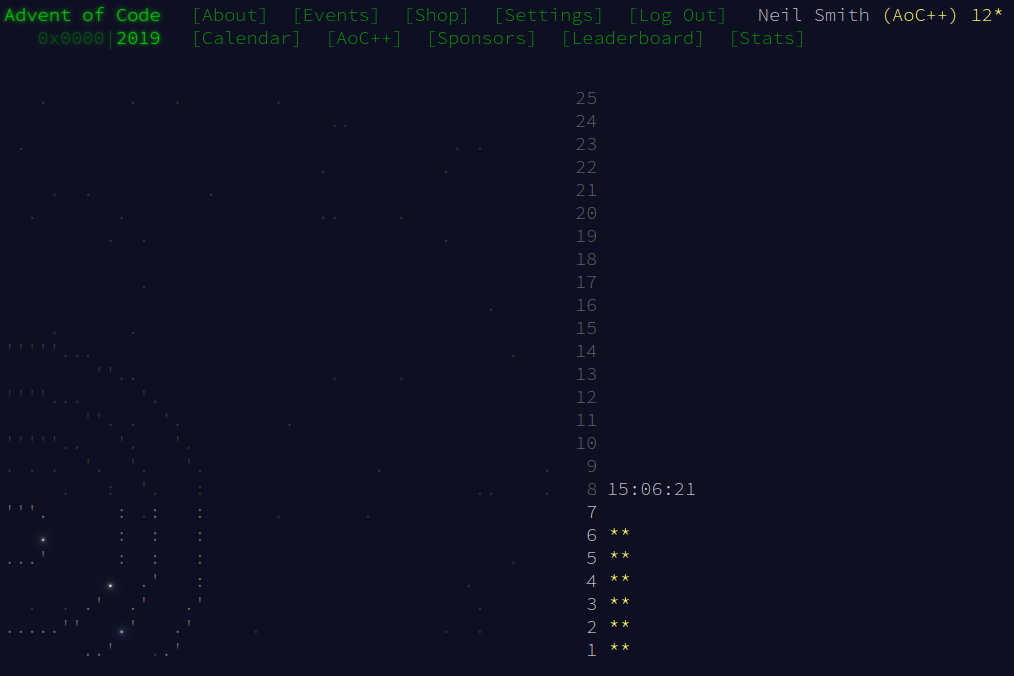December 7, 2019

# Advent of Code 2019 day 6

The joys of not over-thinking the problemThis one turned out to be much simpler than I first thought. A brief reading of the task description had me thinking about trees of nested orbits and trying to fold over them to find all the orbits. But it turns out that all you need to know is how to go from a satellite to its primary.

I store that information in a Map, which I build using a fold over the list of orbits, inserting each satellite-primary pair as I go.

-- from satellite to primary
type Orbits = M.Map String String

buildOrbits :: [(String, String)] -> Orbits

addOrbit :: Orbits -> (String, String) -> Orbits
addOrbit orbits (primary, satellite) = M.insert satellite primary orbits


## Part 1

Counting the direct and indirect orbits for a single satellite is 1, plus the number of orbits available from its primary.

orbitCount :: Orbits -> String -> Int
orbitCount orbits here
| here M.member orbits = 1 + (orbitCount orbits (orbits!here))
| otherwise = 0


If you do that for all the satellites, you get the total number of orbits.

part1 :: Orbits -> Int
part1 orbits = sum $map (orbitCount orbits)$ M.keys orbits


## Part 2

You could do this with a graph-search algorithm. But given that the set of orbits is a tree, an easier way to do it is

1. Find all the steps from YOU to the common primary, COM.
2. Find all the steps from SAN to COM.

Those two paths will meet at some point and be identical from there onwards. Say they meet at SWI, the switching planet. To move from YOU to SAN, you follow the path from YOU to COM until you get to SWI, then the reversed path from SAN to SWI.

You can find the distinct parts of each transfer path with the difference operation. And as you only need the number of steps, you only need the sizes of the paths. That means you can treat the paths as a Set of primaries visited. Because SWI is common to both paths, it will be excluded from the distinct parts, so the sets will be too small; but that's fixed by including the direct primary of YOU and SAN in their respective paths.

And here's the code. transferSteps finds all the primaries visited going from a satellite to COM.

transferSteps :: Orbits -> Transfers -> String -> Transfers
transferSteps orbits transfers here
| here M.member orbits = transferSteps orbits transfers' there
| otherwise = transfers'
where there = orbits!here
transfers' = S.insert here transfers


The code for part2 finds the two paths, finds the distinct parts of them, finds the sizes of those parts, and returns the sum.

part2 :: Orbits -> Int
part2 orbits = youDist + sanDist
where youTrans = transferSteps orbits S.empty (orbits!"YOU")
sanTrans = transferSteps orbits S.empty (orbits!"SAN")
onlyYou = youTrans \\ sanTrans
onlySan = sanTrans \\ youTrans
youDist = S.size onlyYou
sanDist = S.size onlySan


(There's an alternative implementation that uses a Map to keep track of the explicit distances to each step on the path, and then finding the maximal value of distance in the disjoint paths. But for this particular instance, the Set method is simpler.)

## Code

The full solution is on Gitweb (and Github).